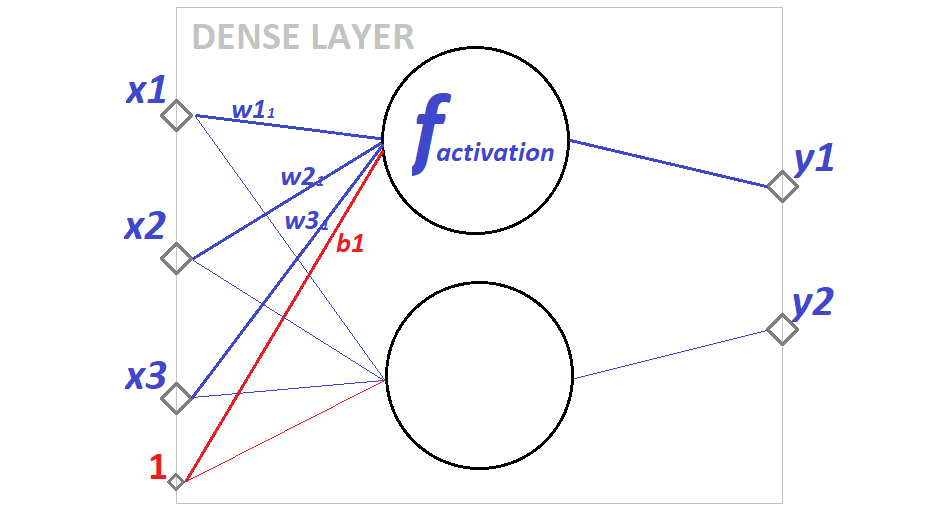# The Basic Building Block of Neural NetworksIn this article, I’m using Keras (https://keras.io/) for exploring layer implementation and source code, but in general, most types of layers are quite generic and the main principles don’t depend that much on the actual library implementing them.

The Dense layer (a regular fully-connected layer) is probably the most widely used and well-known Neural Networks layer. It is the basic building block of many Neural Networks architectures.

Understanding the Dense layer gives a solid base for further exploring other types of layers and more complicated network architectures. Let’s dive into the Dense layer deep down till the code implementing it.

In this article, I’m using Keras (https://keras.io/) for exploring layer implementation and source code, but in general, most types of layers are quite generic and the main principles don’t depend that much on the actual library implementing them.

## Dense layer overview

Let’s start by taking a look at a visual representation of such layer:

In this example, the Dense layer has 3 inputs, 2 units (and outputs) and a bias. Let’s take a look at each of these.

Layer inputs are represented here by x1, x2, x3. This is where data comes in — these can be either input feature values or the output from the previous layer. Technically these can be any numerical values, but in most of the cases, input values will be normalized to an interval of [-1, 1]. Normalization can be done manually or using special layers (e.g. BatchNormalization layer in Keras).

Big circles represent the units. This is where input values are converted to outputs. Outputs are represented by y1 and y2 in the visualization. The number of outputs always matches the number of units. The Dense layer is fully-connected, meaning that it connects every input to every output. This naturally means that every input value affects (or at least can affect — if the corresponding weight value is not zero) each output value.

Conversion from inputs to outputs is defined by the activation function. This function is applied to the input values x1, x2, x3 to obtain output value y. The result of this function is influenced by weights — these are represented as w11, w21, w31 in the visualization. Weights give models the ability to learn — basically what a NN model learns are the values of weights in all layers.

There is one more thing in the visualization — red number “1” with connections to all units, representing the “bias”. The bias specifies some external influence to the output value, which is not covered by features provided in the input.

## Most popular Data Science and Machine Learning courses — July 2020

Most popular Data Science and Machine Learning courses — August 2020. This list was last updated in August 2020 — and will be updated regularly so as to keep it relevant

## Cheat Sheets for AI, Neural Networks, Machine Learning, Deep Learning & Big Data

Cheat Sheets for AI, Neural Networks, Machine Learning, Deep Learning & Big Data

## 15 Machine Learning and Data Science Project Ideas with Datasets

Learning is a new fun in the field of Machine Learning and Data Science. In this article, we’ll be discussing 15 machine learning and data science projects.

## Fundamentals of Neural Network in Machine Learning

Fundamentals of Neural Network in Machine Learning. What is a Neuron? What is the Activation Function? How do Neural Network Works? How do Neural Networks Learn?

## Best Free Datasets for Data Science and Machine Learning Projects

This post will help you in finding different websites where you can easily get free Datasets to practice and develop projects in Data Science and Machine Learning.